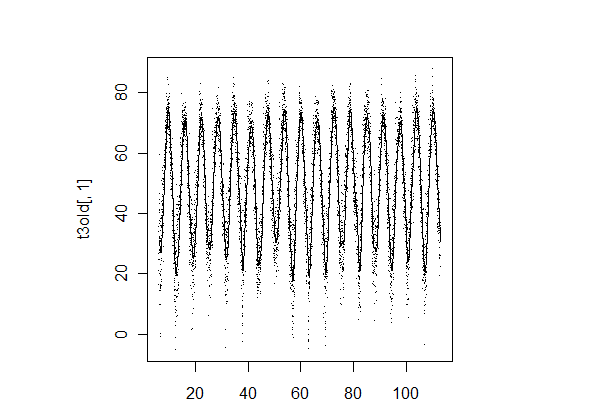## Monday, April 9, 2012

### Example 9.26: More circular plottingSAS's Rick Wicklin showed a simple loess smoother for the temperature data we showed here. Then he came back with a better approach that does away with edge effects. Rick's smoothing was calculated and plotted on a cartesian plane. In this entry we'll explore another option or two for smoothing, and plot the results on the same circular plot.

Since Rick is showing SAS code, and Robert Allison has done the circular plot (plot) (code), we'll stick to the R again for this one.

R
We'll start out by getting the data and setting it up as we did earlier. We add the year back into the matrix t3old because it'll be needed later.
`temp1 = read.table("http://academic.udayton.edu/kissock/http/Weather/           gsod95-current/NYALBANY.txt")leap = c(0,1,0,0,0,1,0,0,0,1,0,0,0,1,0,0,0,1)days = rep(365,18) + leapmonthdays = c(31,28,31,30,31,30,31,31,30,31,30,31)temp1\$V3 = temp1\$V3 - 1994yearpart = function(daytvec,yeardays,mdays=monthdays){  part = (sum(mdays[1:(daytvec-1)],(daytvec > 2) * (yeardays[daytvec]==366))           + daytvec - ((daytvec == 1)*31)) / yeardays[daytvec]  return(part)}temp2 = as.matrix(temp1)radians = 2* pi * apply(temp2, 1, yearpart, days, monthdays)t3old = matrix(c(temp1\$V4[temp1\$V4 != -99 & ((temp1\$V3 < 18) )],                 radians[temp1\$V4 != -99  &  ((temp1\$V3 < 18) )],                 temp1\$V3[temp1\$V4 != -99 & ((temp1\$V3 < 18) )]), ncol=3)t3now= matrix(c(temp1\$V4[temp1\$V4 != -99 & ((temp1\$V3 == 18) |                            (temp1\$V3 == 17 & temp1\$V1 == 12))],                radians[temp1\$V4 != -99 & ((temp1\$V3 == 18) |                            (temp1\$V3 == 17 & temp1\$V1 == 12))]), ncol=2)library(plotrix)radial.plot(t3old[,1],t3old[,2],rp.type="s", point.col = 2, point.symbols=46,     clockwise=TRUE, start = pi/2, label.pos = (1:12)/6 * (pi),    radial.lim=c(-20,10,40,70,100),  labels=c("February 1","March 1",      "April 1","May 1","June 1","July 1","August 1","September 1",      "October 1","November 1","December 1","January 1"))radial.plot(t3now[,1],t3now[,2],rp.type="s", point.col = 1, point.symbols='*',     clockwise=TRUE, start = pi/2, add=TRUE, radial.lim=c(-20,10,40,70,100))`

If you didn't happen to see the update on the previous entry, note that the radial.lim option makes the axes for the added points match those for the initial plot. Otherwise, the added points plotted lower than they appeared, making the recent winter look cooler.

Rick started with a smoother, but often cyclic data can be fit well parametrically, using the sine and cosine of the cycle length as the covariates. With the data set up in radians already, this is trivially simple. The predicted values for the data can be retrieved with the fitted() function (e.g., section 3.7.3), which works with many model objects. These can then be fed into the radial.plot() function with rp.type="p" to make a line plot. The result is shown at the top-- the parametric fit appears to do a good job. Of course, you can fit on a square plot very easily with the plot() function, with result shown below.
`simple = lm(t3old[,1] ~ sin(t3old[,2]) + cos(t3old[,2]))radial.plot(fitted(simple),t3old[,2],rp.type="p", clockwise=TRUE,            start = pi/2, add=TRUE, radial.lim=c(-20,10,40,70,100))plot(t3old[,1] ~ t3old[,2], pch='.')lines(t3old[,2],fitted(simple))`I didn't change the order of the data, so the line comes back to the beginning of the plot at the end of the year.

Adding a smoothed fit is nearly as easy. Just replace the lm() call with a loess() (section 5.2.6) call. The new line is added on top of the old one, to see just how they differ. The result is show below.
`simploess = loess(t3old[,1] ~ t3old[,2])radial.plot(fitted(simploess),t3old[,2],rp.type="p", line.col="blue",           clockwise=TRUE, start = pi/2, add=TRUE, radial.lim=c(-20,10,40,70,100))`The parametric fit is pretty good, but misses the sharp dip seen in January, and the fit in the late fall and early spring appear to be slightly affected.

But this approach stacks up all the data from 18 years. It might be more appropriate to stretch the data across the calendar time, fit the smoothed line to that, and then wrap it around the circular plot. To do this, we'll need to add the year back into the radians. Finding an acceptable smoother was a challenge-- the smooth.spline() function used here was adequate, but as the second plot below shows, it misses some highs and lows. Adding the smoothed curve to the plot is as easy as before, however. The plot with smoothing by year is immediately below.
`radyear = t3old[,2] + (2 * pi * t3old[,3])better = smooth.spline(y=t3old[,1],x= radyear, all.knots=TRUE,spar=1.1)radial.plot(fitted(better),t3old[,2],rp.type="p", line.col="green",         clockwise=TRUE, start = pi/2, add=TRUE, radial.lim=c(-20,10,40,70,100))plot(t3old[,1] ~ radyear, pch = '.')lines(better)`The relatively poor fit seen below makes the new (green) line at least as poor as the parametric fit. The extra variability in the winter is reflected in distinct lines in the winter. Rick's approach, to fit the data lumping across years, seems to be the best for fitting, though it's easier to see the heteroscedaticity in the ciruclar plot. But however you slice it, this winter has had an unusual number of very warm days.An unrelated note about aggregators